# 机器学习中各种熵的定义及理解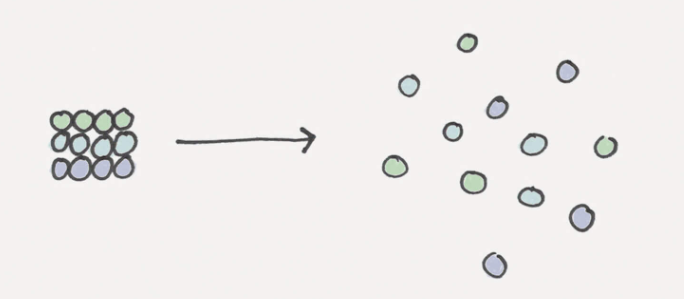## 1. 自信息

“陈羽凡吸毒？！工作室不是刚辟谣了吗？哇！信息量好大！”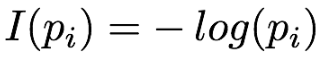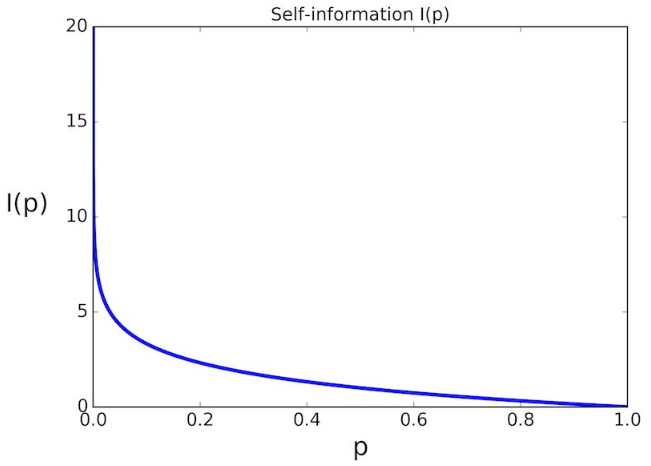## 2. 信息熵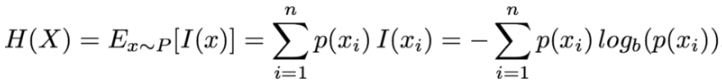H(x)表示用以消除这个事件的不确定性所需要的统计信息量，即信息熵。

 事件 概率 信息量（自信息） 信息熵（统计信息量） 正面朝上 1/2 -log(1/2) (-1/2 * log(1/2))+( -1/2 * log(1/2)) 反面朝上 1/2 -log(1/2) (-1/2 * log(1/2))+( -1/2 * log(1/2))

1. 若事件x个状态发生概率为1，那么信息熵H(x)等于0
2. 若事件x的所有状态n发生概率都一致，即都为1/n，那么信息熵H(x)有极大值logn。## 3. 联合熵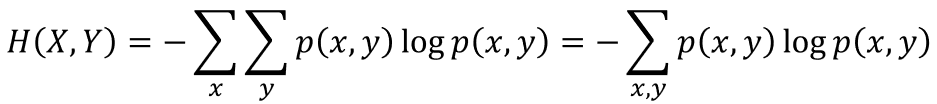事件 概率 信息量（自信息） 联合熵 x正，y正 1/2 * 1/2 = 1/4 -log(1/4) -(1/4*log(1/4)+ 1/4 * log(1/4) + 1/4 * log(1/4) + 1/4 * log(1/4)) x正，y反 1/2 * 1/2 = 1/4 -log(1/4) -(1/4*log(1/4)+ 1/4 * log(1/4) + 1/4 * log(1/4) + 1/4 * log(1/4)) X反，y正 1/2 * 1/2 = 1/4 -log(1/4) -(1/4*log(1/4)+ 1/4 * log(1/4) + 1/4 * log(1/4) + 1/4 * log(1/4)) X反，y反 1/2 * 1/2 = 1/4 -log(1/4) -(1/4*log(1/4)+ 1/4 * log(1/4) + 1/4 * log(1/4) + 1/4 * log(1/4))

## 4. 条件熵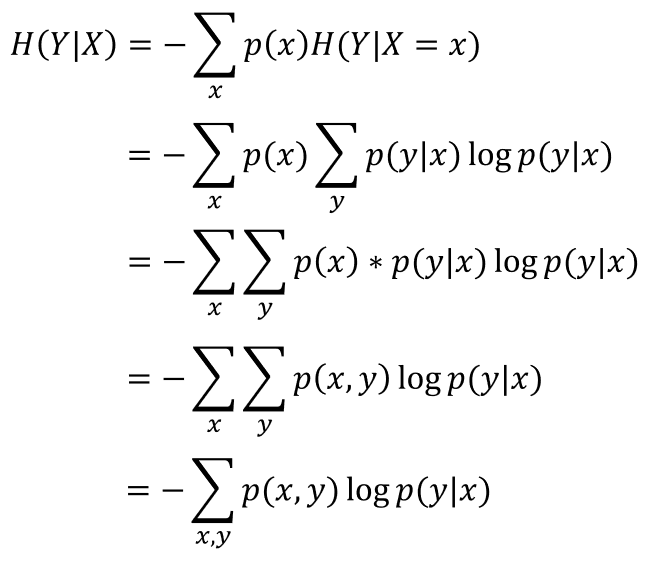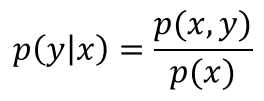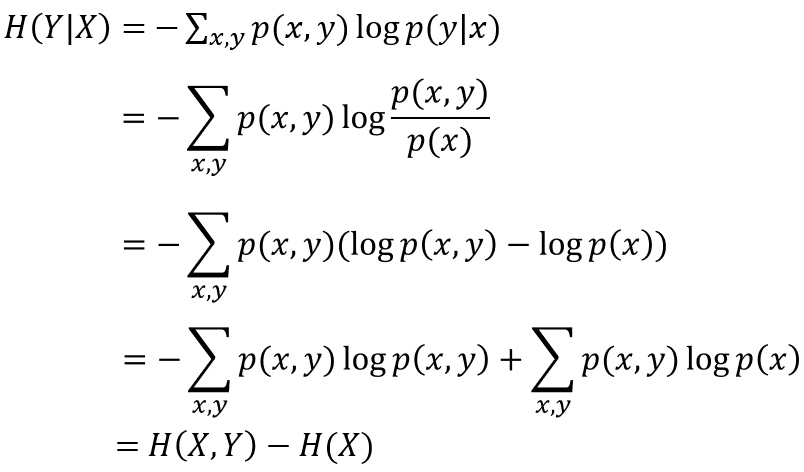## 5. 交叉熵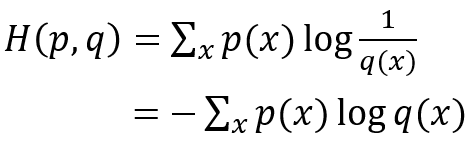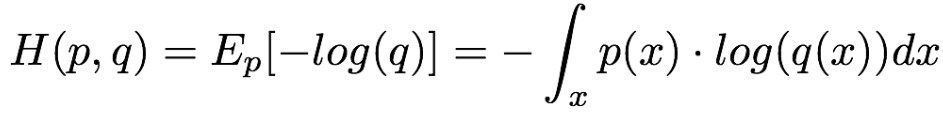## 6. 相对熵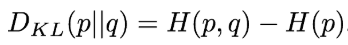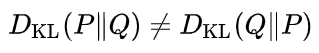## 7. 互信息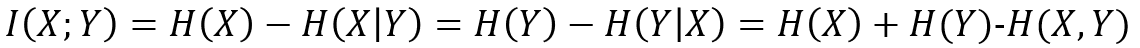## 8. 熵在机器学习中的应用

• 在贝叶斯网络中，会假设一个先验分布，目的是为了反映随机变量在观测前的不确定性。在进行模型训练时，减小熵，同时让后验分布在最可能的参数值周围形成峰值。
• 在做分类任务的参数估计时，尤其是在神经网络中，交叉熵往往作为损失函数用来更新网络权重。
• 在树模型算法中，熵的作用也是不可或缺，尤其是在使用ID3信息增益、C4.5增益率时，通过使用熵来划分子节点，从而可以构造出整棵树。

posted @ 2018-12-06 20:26 Bo_hemian 阅读(...) 评论(...) 编辑 收藏